# Addition of vectors by analytical method

## Vector of Class 11

1. Write the vectors in the form of their components.

2. Add similar components algebraically with proper sign.

3. After addition (with proper sign) they become the components of the resultant vector.

Example: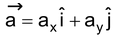,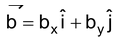and tan α = ay/ax

Illustration 12.Find the net displacement of a particle from its starting point if it undergoes three successive displacements given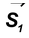= 20 m, 450 West of North,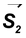= 15 m, 300 North of East;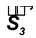=20m, due South.

Solution:Let us set our axial system such that x-axis is along West-East and y-axis along South-North.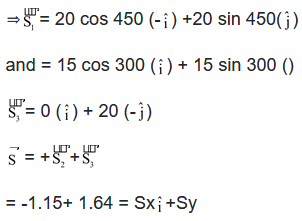= 2m

Direction θ = tan-1 = 350 West of North